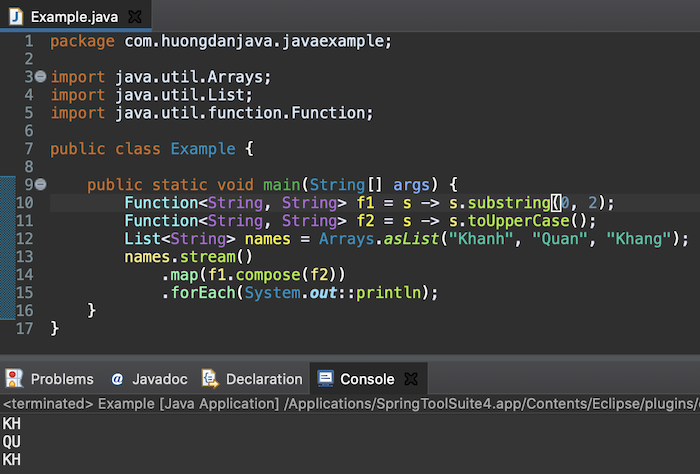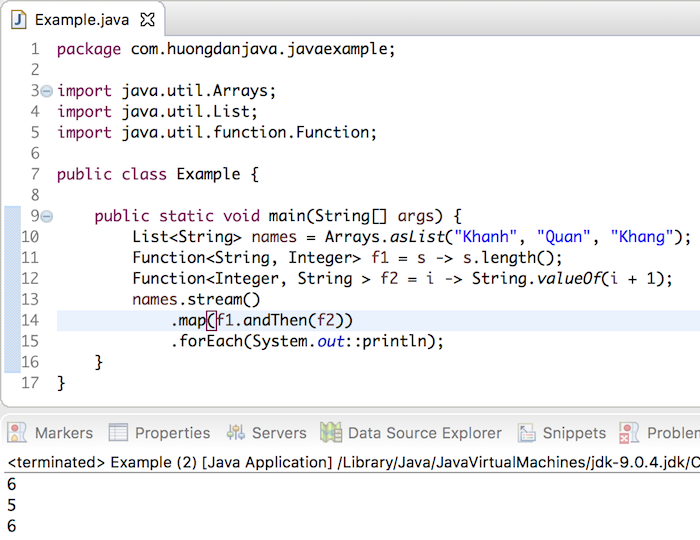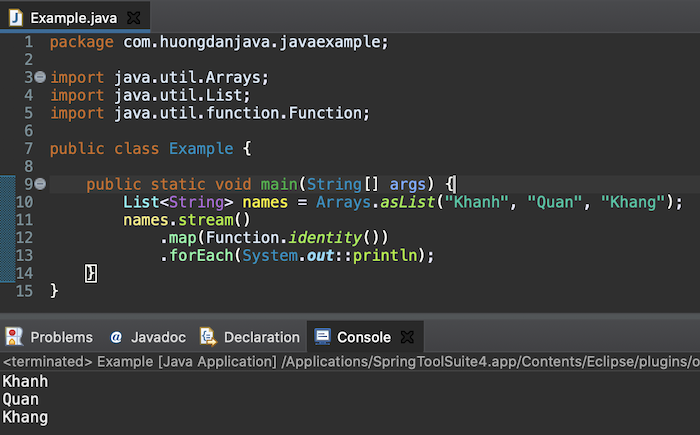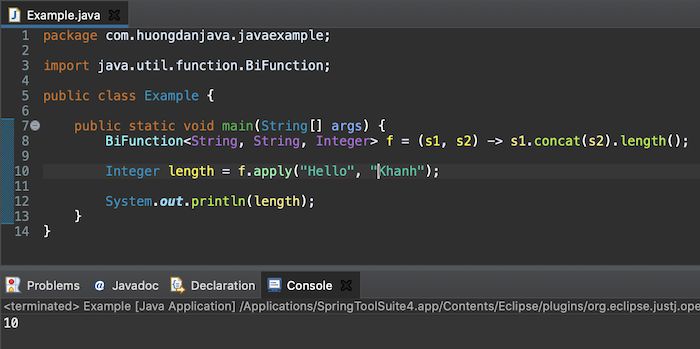# Learn about Function Functional Interface in Java

Function interface is used to convert data from input parameters to return results in a different format. To do this, we will implement the apply() abstract method defined in the Function interface.

But first, let’s look at the contents of the Function interface.

We often use the Function interface in the map() method of the Stream object as the parameter of this method, for example:

As you can see, the Function interface is defined with two generic data types, the first will be the input passed to the parameter of the apply() method and the second will be the output of this apply() method. In my example, the input data is a String data type, and after conversion, the returned data is of type Integer.

We can pass the definition of Function f to the map method as follows:

Result:The compose() method is used to convert many different steps, the result of the next function will be the input of this function. For example:

In the above code, you will see Function f2 will be executed first, then Function f1.

Result:The andThen() method same as compose() method, used to convert with many different steps too, but sequentially, the result of this function will be the input of the next function. For example, after converting a String to Integer, we pass this Integer value to String with a value of 1:

Result:The static identity() method is used to return the value of the input. Whatever value you pass in, the output of this method will be that value. For example:

Result:We have many variants of the Function interface depending on the type of data returned. For example, if we return an Integer we have a ToIntFunction interface or we return a Long, we have the ToLongFunction interface.

BiFunction interface is a variant of Function interface that allows us to pass 2 values to input and return output value, for example:

Here, I define a BiFunction with 2 input String values, output type Integer to return the length of a string after concatenating 2 strings passed in the input.

Result: